Several months ago I wrote a blog post about Lissajous curves and knots that included the image below.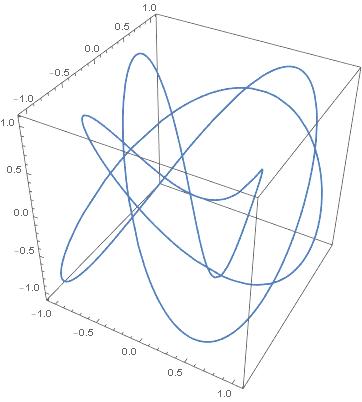Here’s an improved version of the same knot.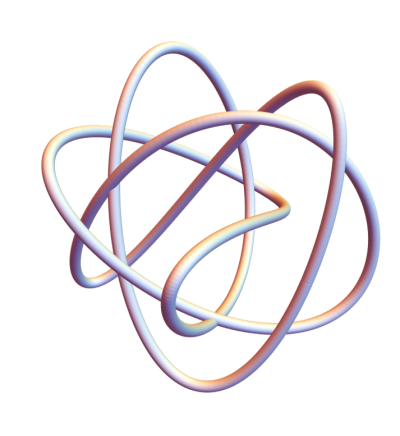The original image was like tying the knot in thread. The new image is like tying it in rope, which makes it easier to see. The key was to use Mathematica’s Tube function to thicken the curve and to see the crossings. I also removed the bounding box in the image.

This was the original code:

    x[t_] := Cos[3 t]
y[t_] := Cos[4 t + Sqrt]
z[t_] := Cos[5 t + Sqrt]

ParametricPlot3D[{x[t], y[t], z[t]}, {t, 0, 2 Pi}]


The new code keeps the definitions of x, y, and z but creates a plot using Tube.


Graphics3D[
Tube[
Table[{x[i], y[i], z[i]}, {i, 0, 2 Pi, 0.01}],
0.05
],
Boxed -> False
]


I went back and changed out the plots in my post Total curvature of a knot with plots similar to the one above.

# A connected topology for the integers

You can define a topology on the positive integers by choosing as an open basis sets the series of the form an + b where a and b are relatively prime positive integers. Solomon Golumb defines this topology in  and proves that it is connected.

But that paper doesn’t prove that proposed basis really is a basis. That is implicitly an exercise for the reader, and we carry out that exercise here. The proof will say that certain things can be computed, and we’ll go a step further an actually compute them with Python.

## Proof

To prove that these sets form the basis of a topology, we need to establish two things.

1. Every point is contained in some basis element.
2. The intersection of basis elements is another basis element or empty.

The first is easy. For any positive number x, the sequence (x+1)n + x contains x, and x+1 is relatively prime to x. Any other number relatively prime to x would work just as well.

The second is more interesting. Consider two sequences: an + b and cm + d. A number x in the intersection satisfies the pair of equations

x = b mod a
x = d mod c.

If a and c are relatively prime, the Chinese Remainder Theorem says that the equation has a solution y, and the solutions are unique mod ac. So the intersection of the two series is acn + y.

If a and c are not relatively prime, let r be their greatest common divisor. Then the intersection of an + b and cm + d is another sequence of the same form if b and d are congruent mod r and empty otherwise.

## Separating points

A topological space is connected if it is not the union of two disjoint open sets. An easy way to make a space connected is to not have any disjoint open sets. But Golumb’s topology has plenty of disjoint open sets.

In fact, his topology is Hausdorff, meaning that any two points can be separated by disjoint open sets. The proof is simple. Take two points s and t. Let p be any prime bigger than both s and t, and consider the two sets pn + s and pn + t.

## Python calculation

Our proof split into two cases: when the strides of the two series are relatively prime and when they are not.

### Relatively prime strides

To get concrete, suppose we have the sequences

2, 9, 16, 23, …

and

3, 14, 25, 35, …

or in other words 7n + 2 and 11n + 3. According to the theory above, these sequences intersect because 7 and 11 are relatively prime, and the intersection should be of the form 77n + y, and we should be able to compute y from the Chinese Remainder Theorem. We will use the function crt from SymPy. (See another example of using this function here.)

    >>> from sympy.ntheory.modular import crt
>>> crt([7,11], [2, 3], symmetric=False)
>>> (58, 77)


This reports that y = 58.

Now let’s verify that the intersection of our two series looks like 77n + 58.

    >>> A = set(2+7*n for n in range(100))
>>> B = set(3+11*n for n in range(100))
>>> sorted(A.intersection(B))
[58, 135, 212, 289, 366, 443, 520, 597, 674]

### Strides with a common factor: empty intersection

Now for the case where a and c have greatest common divisor r > 1. Consider, for example,

1, 16, 31, 46, …

and

2, 14, 26, 38, …

The sequences 15n + 1 and 12m + 2 do not intersect. The greatest common divisor of 15 and 12 is 3. Numbers in the first series are congruent to 1 mod 3 and numbers in the second series are congruent to 2 mod 3, so no number can be in both. If we didn’t realize this, we could call

    crt([15,12], [1, 2], symmetric=False)

and see that it returns None.

### Strides with a common factor: finding the intersection

But now let’s look at

1, 16, 31, 46, …

and

13, 25, 37, 49, …

Now both sequences are congruent to 1 mod 3, so it’s at least feasible that the two series may intersect. And in fact they do.

    >>> crt([15,12], [1, 13], symmetric=False)
(1, 60)


This says that the set of solutions are all congruent to 1 mod 60. Now 1 itself is not a solution, but 61 is, and so is 60n + 1 for all positive integers n. We can illustrate this as before by computing the intersection of the two series.

    >>> A = set(1+15*n for n in range(30))
>>> B = set(13+12*n for n in range(30))
>>> sorted(A.intersection(B))
[61, 121, 181, 241, 301, 361]


## Related posts

 Solomon W. Golomb. A Connected Topology for the Integers. The American Mathematical Monthly , Oct., 1959, pp. 663-665

# Total curvature of a knot

Tie a knot in a rope and join the ends together. At each point in the rope, compute the curvature, i.e. how much the rope bends, and integrate this over the length of the rope. The Fary-Milnor theorem says the result must be greater than 4π. This post will illustrate this theorem by computing numerically integrating the curvature of a knot.

If p and q are relatively prime integers, then the following equations parameterize a knot.

x(t) = cos(pt) (cos(qt) + 2)
y(t) = sin(pt) (cos(qt) + 2)
z(t) = -sin(qt)

This is in fact a torus knot because the curve stays on the surface of a torus (doughnut) . We can get a trefoil knot, for example, by setting p = 2 and q = 3.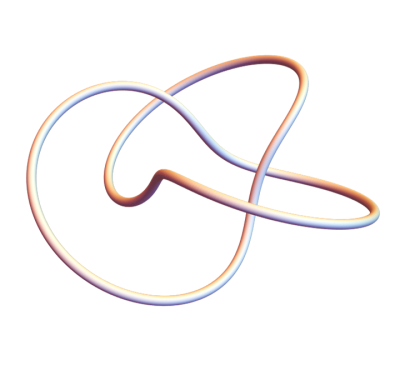We’ll use Mathematica to compute the total curvature of this knot and other examples. First the parameterization:

    x[t_, p_, q_] := Cos[p t] (Cos[q t] + 2)
y[t_, p_, q_] := Sin[p t] (Cos[q t] + 2)
z[t_, p_, q_] := -Sin[q t]


We can plot torus knots as follows.

    k[t_, p_, q_] := {
x[t, p, q],
y[t, p, q],
z[t, p, q]
}
Graphics3D[Tube[Table[k[i, p, q], {i, 0, 2 Pi, 0.01}],
0.08], Boxed -> False]


Here’s a more complicated knot with p = 3 and q = 7.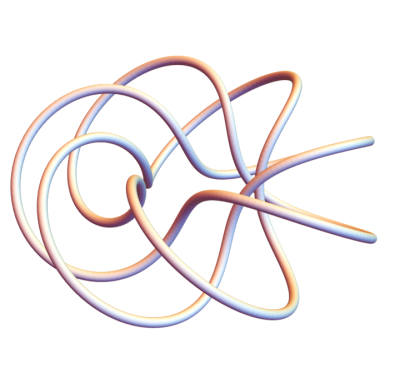Before we can integrate the curvature with respect to arc length, we need an expression for an element of arc length as a function of the parameter t.

    ds[t_, p_, q_] :=  Sqrt[
D[x[t, p, q], t]^2 +
D[y[t, p, q], t]^2 +
D[z[t, p, q], t]^2
]


Now we can compute the total curvature.

    total[p_, q_] := NIntegrate[
ArcCurvature[k[t, p, q], t] ds[t, p, q],
{t, 0, 2 Pi}
]


We can use this to find that the total curvature of the (2,3) torus knot, the trefoil, is 17.8224, whereas 4π is 12.5664. So the Fary-Milnor theorem holds.

Let’s do one more example, this time a (1,4) knot.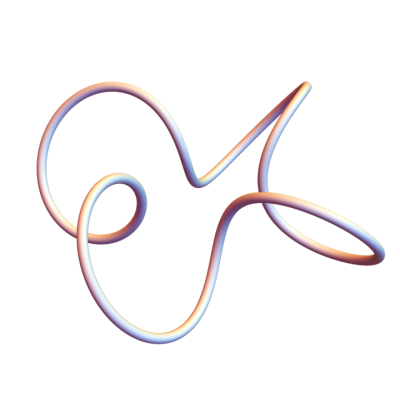You can see that this is not actually knot. This doesn’t contradict what we said above because 1 divides 4. When p or q equal 1, we get an unknot.

When we compute its total curvature we get 24.2737, more than 4π. The Fary-Milnor theorem doesn’t say that total curvature in excess of 4π is a sufficient condition for a loop to be knotted; it says it’s necessary. Total curvature less than 4π proves that something isn’t a knot, but curvature greater than 4π doesn’t prove anything.

## More on curvature and knots

 If you change out the 2s in the parameterization with a larger number, it’s easier to see from the graphs that the curves are on a torus. For example, if we plot the (3,7) knot again, replacing 2’s in the definition of x(t) and y(t) with 5’s, we can kinda see a torus inside the knot.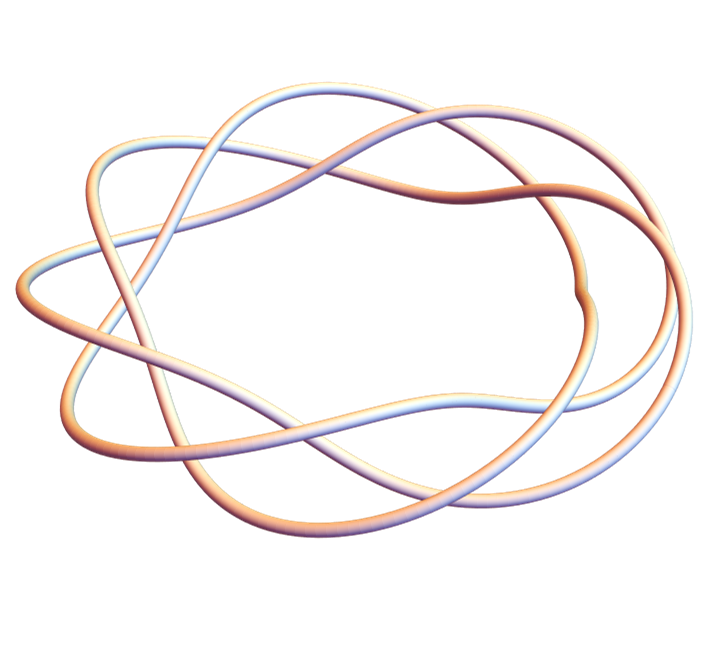# Stone-Weierstrass on a disk

A couple weeks ago I wrote about a sort of paradox, that Weierstrass’ approximation theorem could seem to contradict Morera’s theorem. Weierstrass says that the uniform limit of polynomials can be an arbitrary continuous function, and so may have sharp creases. But Morera’s theorem says that the uniform limit of polynomials is analytic and thus very smooth.

Both are true, but they involve limits in different spaces. Weierstrass’ theorem applies to convergence over a compact interval of the real line, and Morera’s theorem applies to convergence over compact sets in the complex plane. The uniform limit of polynomials is better behaved when it has to be uniform in two dimensions rather than just one.

This post is a sort of variation on the post mentioned above, again comparing convergence over the real line and convergence in the complex plane, this time looking at what happens when you conjugate variables .

There’s an abstract version of Weierstrass’ theorem due to Marshall Stone known as the Stone-Weierstrass theorem. It generalizes the real interval of Weierstrass’ theorem to any compact Hausdorff space . The compact Hausdorff space we care about for this post is the unit disk in the complex plane.

There are two versions of the Stone-Weierstrass theorem, one for real-valued functions and one for complex-valued functions. I’ll state the theorems below for those who are interested, but my primary interest here is a corollary to the complex Stone-Weierstrass theorem. It says that any continuous complex-valued function on the unit disk can be approximated as closely as you like with polynomials in z and the conjugate of z with complex coefficients, i.e. by polynomials of the formWhen you throw in conjugates, things change a lot. The uniform limit of polynomials in z alone must be analytic, very well behaved. But the uniform limit of polynomials in z and z conjugate is merely continuous. It can have all kinds of sharp edges etc.

Conjugation opens up a lot of new possibilities, for better or for worse. As I wrote about here, an analytic function can only do two things to a tiny disk:  stretch it or rotate it. It cannot flip it over, as conjugation does.

By adding or subtracting a variable and its conjugate, you can separate out the real and imaginary parts. The the parts are no longer inextricably linked and this allows much more general functions. The magic of complex analysis, the set of theorems that seem too good to be true, depends on the real and imaginary parts being tightly coupled.

***

Now for those who are interested, the statement of the Stone-Weierstrass theorems.

Let X be a compact Hausdorff space. The set of real or complex valued functions on X forms an algebra. That is, it is closed under taking linear combinations and products of its elements.

The real Stone-Weierstrass theorem says a subalgebra of the continuous real-valued functions on X is dense if it contains a non-zero constant function and if it separates points. The latter condition means that for any two points, you can find functions in the subalgebra that take on different values on the two points.

If we take the interval [0, 1] as our Hausdorff space, the Stone-Weierstrass theorem says that the subalgebra of polynomials is dense.

The complex Stone-Weierstrass theorem says a subalgebra of he continuous complex-valued functions on X is dense if it contains a non-zero constant function, separates points, and is closed under taking conjugates. The statement above about polynomials in z and z conjugate follows immediately.

***

 For a complex variable z of the form a + bi where a and b are real numbers and i is the imaginary unit, the conjugate is abi.

 A Hausdorff space is a general topological space. All it requires is that for any two distinct points in the space, you can find disjoint open sets that each contain one of the points.

In many cases, a Hausdorff space is the most general setting where you can work without running into difficulties, especially if it is compact. You can often prove something under much stronger assumptions, then reuse the proof to prove the same result in a (compact) Hausdorff space.

See this diagram of 34 kinds of topological spaces, moving from strongest assumptions at the top to weakest at the bottom. Hausdorff is almost on bottom. The only thing weaker on that diagram is T1, also called a Fréchet space.

In a T1 space, you can separate points, but not simultaneously. That is, given two points p1 and p2, you can find an open set U1 around p1 that does not contain p2, and you can find an open set U2 around p2 that does not contain p1. But you cannot necessarily find these sets in such a way that U1 and U2 are disjoint. If you could always choose these open sets to be distinct, you’d have a Hausdorff space.

Here’s an example of a space that is T1 but not Hausdorff. Let X be an infinite set with the cofinite topology. That is, open sets are those sets whose complements are finite. The complement of p1 is an open set containing p2 and vice versa. But you cannot find disjoint open sets separating two points because there are no disjoint open sets. The intersection of any pair of open sets must contain an infinite number of points.

# Putting topological data analysis in context

I got a review copy of The Mathematics of Data recently. Five of the six chapters are relatively conventional, a mixture of topics in numerical linear algebra, optimization, and probability. The final chapter, written by Robert Ghrist, is entitled Homological Algebra and Data. Those who grew up with Sesame Street may recall the song “Which one of these, is not like the other …”

When I first heard of topological data analysis (TDA), I was excited about the possibility of putting some beautiful mathematics to practical application. But it was hard for me to put TDA in context. How do you get actionable information out of it? If you find a seven-dimensional doughnut hiding in your data, that’s very interesting, but what do you do with that information?

Robert’s chapter in the book I’m reviewing has a nice introductory paragraph that helps put TDA in context. The section heading for the paragraph is “When is Homology Useful?”

Homological methods are, almost by definition, robust, relying on neither precise coordinates nor careful estimates for efficiency. As such, they are most useful in settings where geometric precision fails. With great robustness comes both great flexibility and great weakness. Topological data analysis is more fundamental than revolutionary: such techniques are not intended to supplant analytic, probabilistic, or spectral techniques. They can however reveal a deeper basis for why some data sets and systems behave the way they do. It is unwise to wield topological techniques in isolation, assuming that the weapons of unfamiliar “higher” mathematics are clad with incorruptible silver.

Robert’s background was in engineering and more conventional applied mathematics before he turned to applications of topology, and so he brings a broader perspective to TDA than someone trained in topology looking for ways to make topology useful. He also has a decade more experience applying TDA than when I interviewed him here. I’m looking forward to reading his new chapter carefully.

As I wrote about the other day, apparently the US Army believes that topological data analysis can be useful, presumably in combination with more quantitative methods.  More generally, it seems the Army is interested in mathematical models that are complementary to traditional models, models that are robust and flexible. The quote above cautions that with robustness and flexibility comes weakness, though ideally weakness that is offset by other models.

## More on topological data analysis

 Algebraic topology is quantitative in one sense and qualitative in another. It aims to describe qualitative properties using algebraic invariants. It’s quantitative in the sense of computing homology groups, but it’s not as directly quantitative as more traditional mathematical models. It’s quantitative at a higher level of abstraction.

# A genius can admit finding things difficult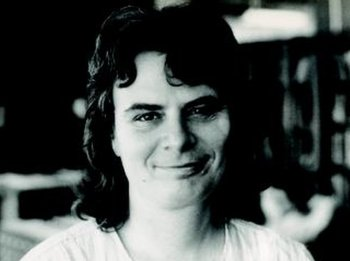Karen Uhlenbeck has just received the Abel Prize. Many say that the Fields Medal is the analog of the Nobel Prize for mathematics, but others say that the Abel Prize is a better analog. The Abel prize is a recognition of achievement over a career whereas the Fields Medal is only awarded for work done before age 40.

I had a course from Karen Uhlenbeck in graduate school. She was obviously brilliant, but what I remember most from the class was her candor about things she didn’t understand. She was already famous at the time, having won a MacArthur genius award and other honors, so she didn’t have to prove herself.

When she presented the definition of a manifold, she made an offhand comment that it took her a month to really understand that definition when she was a student. She obviously understands manifolds now, having spent her career working with them.

I found her comment about extremely encouraging. It shows it’s possible to become an expert in something you don’t immediately grasp, even if it takes you weeks to grok its most fundamental concept.

Uhlenbeck wasn’t just candid about things she found difficult in the past. She was also candid about things she found difficult at the time. She would grumble in the middle of a lecture things like “I can never remember this.” She was not a polished lecturer—far from it—but she was inspiring.

Photo of Karen Uhlenbeck in 1982 by George Bergman [GFDL], via Wikimedia Commons

# Summarizing homotopy groups of spheres

I don’t understand homotopy groups of spheres, and it’s OK if you don’t either. Nobody fully understands them. This post is really more about information compression than homotopy. That is, I’ll be looking at ways to summarize what is known without being overly concerned about what the results mean.

## The task: map two integers to a list of integers

For each positive integer k, and non-negative integer n, the kth homotopy group of the sphere Sn is a finitely generated Abelian group, something that can be described by a finite list of numbers. So we’re looking at simply writing a function that takes two integers as input and returns a list of integers. This function is implemented in an online calculator that lets you lookup homotopy groups.

Computing homotopy groups of spheres is far from easy. The first Fields medal given to a topologist was for partial work along these lines. There are still groups that haven’t been computed, and potentially more Fields medals to win. But our task is much more modest: simply to summarize what has been discovered.

This is not going to be too easy, as suggested by the sample of results in the table below.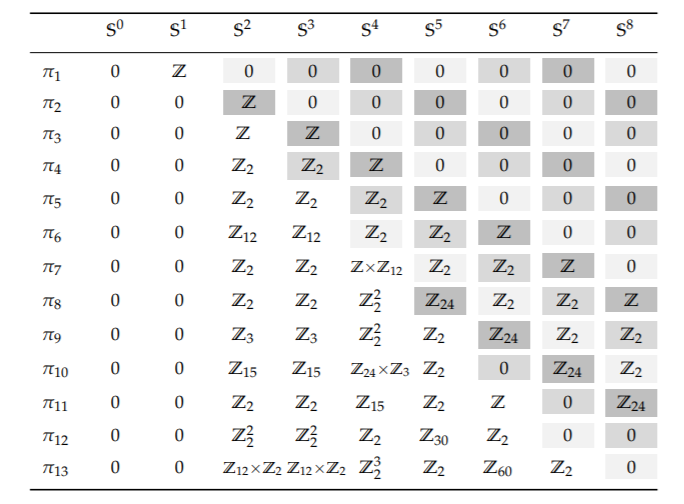This table was taken from the Homotopy Type Theory book, and was in turn based on the Wikipedia article on homotopy groups of spheres.

## Output data representation

To give an example of what we’re after, the table says that π13(S²), the 13th homotopy group of the 2-sphere, is Z12 × Z2. All we need to know is the subscripts on the Z‘s, the orders of the cyclic factors, and so our function would take as input (13, 2) and return (12, 2).

The table tells us that π8(S4) = Z22. This is another way of writing Z2 × Z2, and so our function would take (8, 4) as input and return (2, 2).

When I said above that our function would return a list of integers I glossed over one thing: some of the Z‘s don’t have a subscript. That is some of the factors are the group of integers, not the group of integers mod some finite number. So we need to add an extra symbol to indicate a factor with no subscript. I’ll use ∞ because the integers as the infinite cyclic group. For example, our function would take (7, 4) and return (∞, 12). I will also use 1 to denote the trivial group, the group with 1 element.

Some results are unknown, and so we’ll return an empty list for these.

The order of the numbers in the output doesn’t matter, but we will list the numbers in descending order because that appears to be conventional.

## Theorems

Some of the values of our function can be filled in by a general theorem, and some will simply be data.

If we call our function f, then there is a theorem that says f(kn) = (1) if kn.  This accounts for the zeros in the upper right corner of the chart above.

There’s another theorem that says f(n+mn) is independent of n if n > m + 1. These are the so-called stable homotopy groups.

The rest of the groups are erratic; we can’t do much better than just listing them as data.

(By the way, the corresponding results for homology rather than homotopy are ridiculously simple by comparison. For k > 0, the kth homology group of Sn is isomorphic to the integers if k = n and is trivial otherwise.)

# Most useful math class

A few years ago someone asked me what was my most useful undergraduate math class. My first thought was topology.

I have never directly applied topology for a client. Nobody has ever approached me wanting to know, for example, whether two objects were in the same homotopy class. But I believe topology was one of the most important classes I took for three reasons.

First, I learned how to prove things in that course. It was a small, interactive class with an excellent teacher (Jim Vick). I might have learned the same techniques in a different class, but for me I learned them in topology.

Second, the course built my confidence. I was apprehensive about taking the course because I knew nothing about it. The little I’d heard about topology—stretching coffee cups into donuts etc.—made me wonder what a class could possibly be like. I proved to myself that I could jump into something unfamiliar and do well.

Finally, the course gave me a solid foundation for analysis, and analysis I have applied more directly. I got a thorough understanding of foundational ideas like continuity and compactness, and a foretaste of measure theory. The course also provided my first brief exposure to category theory. To this day, my Pavlovian response to a mention of functors is to think of the fundamental group of a topological space.

I look back on topology the way many look back on a classical education, something not directly useful but indirectly very useful.

# Agile software development and homotopy

One of the things I learned from my tenure as a software project manager was that a project is more likely to succeed if there’s a way to get where you want to go continuously. You want to move a project from A to B gradually, keeping a working code base all along the way. At the end of each day, the software may not be fully functional, but it should at least build. Anything that requires a big bang change, tearing the system apart for several days and putting it back together, is less likely to succeed.

This is very much like the idea of homotopy from topology, a continuous deformation of one thing into another. No discontinuities along the way—no ripping, no jumping suddenly from one thing to another.

# Next areas of math to be applied

Not that long ago number theory was considered strictly pure math. Then came applications to cryptography. Now number theory is at the foundation of the online economy.

What are the next areas of pure math to find widespread application? Some people are saying algebraic topology and category theory.

[I saw a cartoon to this effect the other day but I can’t find it. If I remember correctly, someone was standing on a hill labeled “algebraic topology” and looking over at hills in the distance labeled with traditional areas of applied math. Differential equations, Fourier analysis, or things like that. If anybody can find that cartoon, please let me know.]

## Algebraic topology

The big idea behind algebraic topology is to turn topological problems, which are hard, into algebraic problems, which are easier. For example, you can associate a group with a space, the fundamental group, by looking at equivalence classes of loops. If two spaces have different fundamental groups, they can’t be topologically equivalent. The converse generally isn’t true: having the same fundamental group does not prove two spaces are equivalent. There’s some loss of information going from topology to algebra, which is a good thing. As long as information you need isn’t lost, you get a simpler problem to work with.

Fundamental groups are easy to visualize, but hard to compute. Fundamental groups are the lowest dimensional case of homotopy groups, and higher dimensional homotopy groups are even harder to compute. Homology groups, on the other hand, are a little harder to visualize but much easier to compute. Applied topology, at least at this point, is applied algebraic topology, and more specifically applied homology because homology is practical to compute.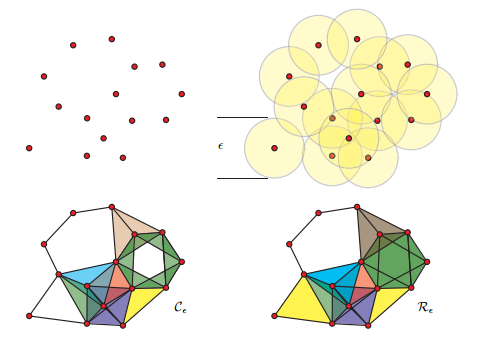People like Robert Ghrist are using homology to study, among other things, sensor networks. You start with a point cloud, such as the location of sensors, and thicken the points until they fuse into spaces that have interesting homology. This is the basic idea of persistent homology.  You’re looking for homology that persists over some range of thickening. As the amount of thickening increases, you may go through different ranges with different topology. The homology of these spaces tells you something about the structure of the underlying problem. This information might then be used as features in a machine learning algorithm. Topological invariants might prove to be useful features for classification or clustering, for example.

Most applications of topology that I’ve seen have used persistent homology. But there may be entirely different ways to apply algebraic topology that no one is looking at yet.

## Category theory

Category theory has been getting a lot of buzz, especially in computer science. One of the first ideas in category theory is to focus on how objects interact with each other, not on their internal structure. This should sound very familiar to computer scientists: focus on interface, not implementation. That suggests that category theory might be useful in computer science. Sometimes the connection between category theory and computer science is quite explicit, as in functional programming. Haskell, for example, has several ideas from category theory explicit in the language: monads, natural transformations, etc.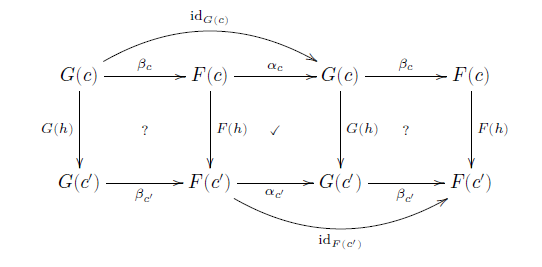Outside of computer science, applications of category theory are less direct. Category theory can guide you to ask the right questions, and to avoid common errors. The mathematical term “category” was borrowed from philosophy for good reason. Mathematicians seek to avoid categorical errors, just as Aristotle and Kant did. I think of category theory as analogous to dimensional analysis in engineering or type checking in software development, a tool for finding and avoiding errors.

I used to be very skeptical of applications of category theory. I’m still skeptical, though not as much. I’ve seen category theory used as a smoke screen, and I’ve seen it put to real use. More about my experience with category theory here.

* * *

Topology illustration from Barcodes: The persistent topology of data by Robert Ghrist.

Category theory diagram from Category theory for scientists by David Spivak